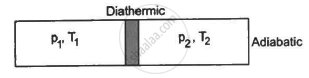Department of Pre-University Education, KarnatakaPUC Karnataka Science Class 11
Advertisement Remove all ads

# Figure Shows a Cylindrical Tube of Volume V with Adiabatic Walls Containing an Ideal Gas. the Internal Energy of this Ideal Gas is Given by 1.5 Nrt. - Physics

Sum

Figure shows a cylindrical tube of volume V with adiabatic walls containing an ideal gas. The internal energy of this ideal gas is given by 1.5 nRT. The tube is divided into two equal parts by a fixed diathermic wall. Initially, the pressure and the temperature are p1, T1 on the left and p2, T2 on the right. The system is left for sufficient time so that the temperature becomes equal on the two sides. (a) How much work has been done by the gas on the left part? (b) Find the final pressures on the two sides. (c) Find the final equilibrium temperature. (d) How much heat has flown from the gas on the right to the gas on the left?Advertisement Remove all ads

#### Solution

Let n1 , U1 and n2 ,U2 be the no. of moles , internal energy of ideal gas in the left chamber and right chamber respectively.

(a) As the diathermic wall is fixed, so final volume of the chambers will be same. Thus, ΔV = 0, hence work done ΔW= P Δ V = 0

by eq. of state in the first and second chamber

P_1V/2=n_1RT_1

rArr n_1=(P_1V)/(2RT_1)

P_2V/2=n_2RT_2

rArr n_2=(P_2V)/(2RT_2)

n = n1 + n2

rArrn=(P_1V)/(2RT_1)+(P_2V)/(2RT_2)=V/(2R)((P_1T_2+P_2T_1)/(T_1T_2))

Again,

U = nCvT

rArrnC_"v"T=1.5nRT

rArrC_"v"=1.5R

In the first and second chamber internal energy is given by

U1 = n1CvT1 = n1 1.5RT1

U2 = n2CvT2 = n2 1.5RT2

U = U1 + U2

1.5nRT = n1 1.5RT1 + n2 1.5RT2

⇒ nT = n1T1 + n2T2

rArrnT = (P_1V)/(2RT_1)T_1+(P-2V)/(2RT_2)T_2=((P_1+P_2)V)/(2R)

rArrT=((P_1+P_2)V)/(2nR)=((P_1+P_2)V)/(2RV/(2R)((P_1T_2+P_2T_1)/(T_1T_2)))=(T_1T_2(P_1+P_2))/(P_1T_2+P_2T_1)................(1)

b) Let final pressure in the first and second compartment P1' and P2'.

By five variable equ of state in the first chamber

(P_1V/2)/T_1=(P_1'V/2)/T

rArrP_1'=P_1/T_1T

By eq. (1)

rArrP_1'=P_1/T_1(T_1T_2(P_1+P_2))/(P_1T_2+P_2T_1)=(P_1T_2(P_1+P_2))/(P_1T_2+P_2T_1)

Similarly,

rArrP_1'=P_2/T_2T=(P_2T_1(P_1+P_2))/(P_1T_2+P_2T_1)

c) Final temperature will be

T=(T_1T_2(P_1+P_2))/(P_1T_2+P_2T_1).............\left(\text{by equation (1)}\right)

d) Heat lost by right chamber will be

n2CvT2 - n2CvT

=(P_2V)/(2RT_2)1.5RT_2-(P_2V)/(2RT_2)1.5R(T_1T_2(P_1+P_2))/(P_1T_2+P_2T_1)

=(3P_2V)/4-(3P_2V)/4(T_1(P_1+P_2))/(P_1T_2+P_2T_1)

=(3P_2V)/4[1-(T_1(P_1+P_2))/(P_1T_2+P_2T_1)]

=(3P_2V)/4[(P_1T_2+P_2T_1-T_1(P_1+P_2))/(P_1T_2+P_2T_1)]

=(3P_1P_2V)/4[(T_2-T_1)/(P_1T_2+P_2T_1)]

Concept: Heat, Internal Energy and Work
Is there an error in this question or solution?
Advertisement Remove all ads

#### APPEARS IN

HC Verma Class 11, Class 12 Concepts of Physics Vol. 2
Chapter 4 Laws of Thermodynamics
Q 21 | Page 64
Advertisement Remove all ads
Advertisement Remove all ads
Share
Notifications

View all notifications

Forgot password?